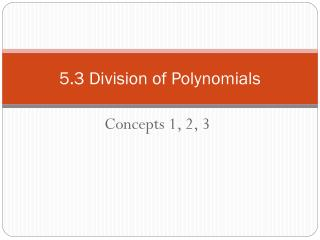DownloadDownload Presentation5.3 Division of Polynomials

# 5.3 Division of Polynomials

Download Presentation## 5.3 Division of Polynomials

- - - - - - - - - - - - - - - - - - - - - - - - - - - E N D - - - - - - - - - - - - - - - - - - - - - - - - - - -
##### Presentation Transcript

1. 5.3 Division of Polynomials Concepts 1, 2, 3

2. 5.3.1 Division by a Monomial • Example:

3. Divide the polynomials.

5. What if you are dividing by a polynomial? • The last method we did won’t work. • There are three ways to do this: • LONG DIVISION • SYNTHETIC DIVISION • FACTORING (we will cover next week)

6. 5.3.2 Long Division • Number example • How do we divide 867 by four using long division? • Similar when using polynomials?

7. Divide the polynomial using long division. CHECK: (divisor)(quotient)+(remainder) = what was divided

8. Divide the polynomial using long division.

9. Divide the polynomial using long division.

10. Divide the polynomial using long division.

11. Divide the polynomial using long division.

12. Divide the polynomial using long division.

13. 5.3.3 Synthetic Division • It is a shortcut way of doing long division. • Only works when divided by something in the form x – c • Set-up: • Degree is always one less than the original

14. Divide the polynomial using synthetic division.

15. Divide the polynomial using synthetic division.

16. Divide the polynomial using synthetic division.

17. Divide the polynomial using synthetic division.

18. HOMEWORK FOR 5.3 • Pg. 338 – 340 • #10, 24, 32, 37, 44, 57